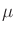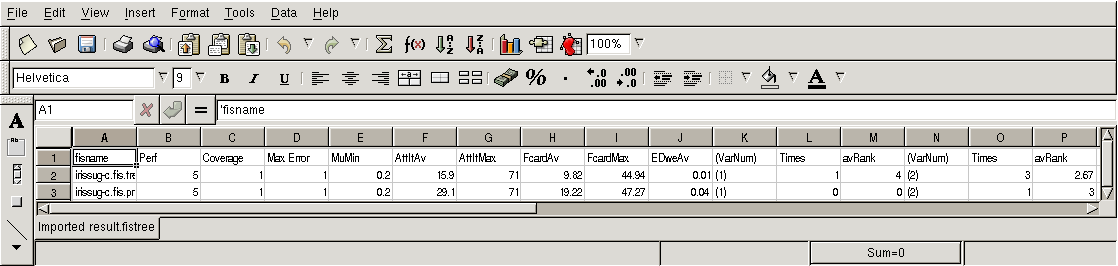Fuzzy decision trees are an extension of classical decision trees [1,14]. They are formed of one root node, which is the tree top or starting point, and a series of other nodes. Terminal nodes are called leaf nodes, or leaves. Each node corresponds to a split on the values of one input variable. This variable is chosen in order to reach a maximum of homogeneity amongst the examples that belong to the node, relatively to the output variable (response variable). This is equivalent to minimizing the entropy. The paths from the root node towards the leaf nodes are easy to interpret as decision rules, strict of fuzzy depending on the nature of the tree.

The tree can be pruned, by transforming a node into a leaf node, if the performance loss is low. This procedure facilitates the tree interpretation. Pruning is based on the performance of the tree equivalent FIS.

Note: the pruned tree may have a better performance than the full tree. as tree building is based on a purity criterion and not directly on a performance criterion.

Fuzzy decision trees proposed in FisPro are based on a fuzzy implementation of the ID3 algorithm .

Generation

The fuzzy decision tree generation procedure in FisPro needs both a current FIS configuration file and a current data file. The tree building is based on one output only, even if the FIS has several outputs. This output is user chosen.

Output Type

Four cases are possible:

• fuzzy output, with the classification option
• fuzzy output, without the classification option
• crisp output, with the classification option
• crisp output, without the classification option (regression tree).
The first three cases use the fuzzy entropy criterion to build the tree, the last one uses a deviance criterion, based on the output value dispersion at each node and is better suited to a regression case.

For a crisp output with the classification option, classes are determined from data, and discrete MFs corresponding to the class labels are assigned to the output. The maximum number of classes is 100.

With the classification option, the majority class is assigned to each node, without it, the average of the node attracted example output is assigned to it.

In the fuzzy output case, the tree summary gives the fuzzy proportions of the node attracted example output for each MF.

Rules

If rules are present in the FIS configuration file, they are ignored.

Options

The Generate tree option opens a pop up windows allowing to choose:

• the tree file name. The tree file format is a description suited for viewing the tree (extension .tree),
• the maximum tree depth, default is the number of variables.
• the minimum matching degreefor an item to be considered as belonging to the node (for entropy calculation),
• The minimum number of samples attracted by a node, with a node membership greater than, required for further splitting the node,
• the tolerance on the matching degree to the node majority class,
• the minimum entropy/deviance gain required (relative value) to build a tree branch
• for classification only, the entropy criterion for building the full tree: absolute (default) or relative entropy gain.
• for regression only, it is possible to optimize rule conclusions in a second pass, using the least square optimization (OLS).

The relative entropy favors the variables with an unequal distribution of examples (not classes) between the MFs. It also favors the variables with a small number of MFs.

• the pruning option:

Pruning consists in a recursive node removal, from tree bottom to top. The removal is done if the equivalent FIS performance does not decrease, or decreases only a little.

The relative loss of performance (compared to the performance of the full tree) has a default value of 0.1. It is user editable. The default validation file used for computing the performance is the current data file. Other files can be used, in particular the active or inactive data file created by the use of the Data-table menu option.

Pruning can be done by removing a full split, or by removing node after node.

• an intermediate output display.

Output

The procedure creates one tree or two (if pruning was selected). It opens two windows for each tree:

• a viewing window.

The user can select the informations to display for all nodes: number of items attracted by the node, node entropy, majority class and sample number class distribution (classification case), or else mean and standard deviation (regression case). The part of the tree displayed in the window can be exported or printed.

The initial tree location is done automatically but the user can also select tree branches and maually move them to improve the location. The scale and font are editable.

• a FIS window. It displays a FIS equivalent to the generated fuzzy tree, which can then be used as such and saved.
• a result file, called result.fistree (figure 3):Each line in the result.fistree file describes a fuzzy tree (full or pruned tree): The first column gives the FIS equivalent name (full tree or prunded tree). Then we have the indices described in section 2:

2nd column: performance index
3rd column: coverage index
4th column: max error
5th column: membership threshold for computing the coverage index

Then follow some indices specific to fuzzy trees:

6th column: average number of examples attracted by a leaf
7th column: maximum number of examples attracted by a leaf
8th column: average fuzzy cardinality per leaf
9th column: maximum fuzzy cardinality for the leaves
10th column: average entropy or deviance per leaf, weighted by the fuzzy cardinality
groups of 3 columns (number of groups equal to number of active FIS input variables)

• variable number
• number of times when it was chosen as the split variable
• average appearing rank

following columns: rule base characteristics (see section 3).

View tree

This submenu allows to view an existing tree. Two possibilities are offered.

Graph: the viewing window described above.
Table: non graphical viewing, displaying the same information in a table.
It opens the viewing window described above.